# Texas Go Math Grade 5 Lesson 3.6 Answer Key Decimal Multiplication

Refer to our Texas Go Math Grade 5 Answer Key Pdf to score good marks in the exams.
Test yourself by practicing the problems from

## Texas Go Math Grade 5 Lesson 3.6 Answer Key Decimal Multiplication

Investigate

Materials; color pencils

The distance from Charlene’s house to her school is 0.8 mile.
Charlene rides her bike 7 tenths of the distance and walks the rest of the way.
How far does Charlene ride her bike to school?

You can use a decimal square to multiply decimals.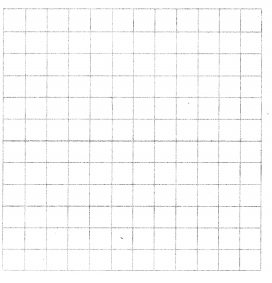Multiply. 0.7 × 0.8
A. Draw a square with 10 equal columns.
What decimal value does each column represent? ___0.1_______

B. Using a color pencil, shade columns on the grid to represent the distance to Charlene’s school.
The distance to the school is 0.8 mile.
How many columns did you shade?____8______

C. Divide the square into 10 equal rows.
What decimal value does each row represent? ___0.1_______

D. Using a different color, shade rows that overlap the shaded columns to represent the distance to school that Charlene rides her bike.

• What part of the distance to school does Charlene ride her bike? _____0.56_____
• How many rows of the shaded columns did you shade? _____7_____

E. Count the number of squares that you shaded twice.
There are _____56_____ squares. Each square represents ____0.01______.
Record the value of the squares as the product. 0.7 × 0.8 = ___0.56_______

So, Charlene rides her bike for ___0.56______ mile.Charlene rides her bike for 0.56 miles,

Explanation:
A. Drawn a square with 10 equal columns as shown above,
The decimal value does each column represent is 0.1,
B. Using a color pencil, shade columns on the grid
to represent the distance to Charlene’s school,
The distance to the school is 0.8 mile, So columns shaded are 8,
C. Divided the square into 10 equal rows,
The decimal value does each row represent is 0.1,
D. Using a different color, shade rows that overlap
the shaded columns to represent the distance to school that Charlene rides her bike.

• The distance to school does Charlene ride her bike 0.56,
• Number of rows of the shaded columns did he shade is 7.

Make Connections

You can use decimal squares to multiply decimals greater than 1.

Multiply. 0.3 × 1.4
STEP 1:
Shade columns to represent 1.4. How many tenths are in 1.4?

STEP 2:

STEP 3:
Count the number of squares that you shaded twice. Record the product at the right.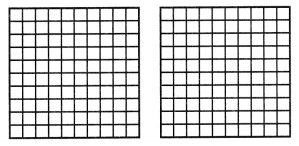0.3 × 1.4 = ______0.42_______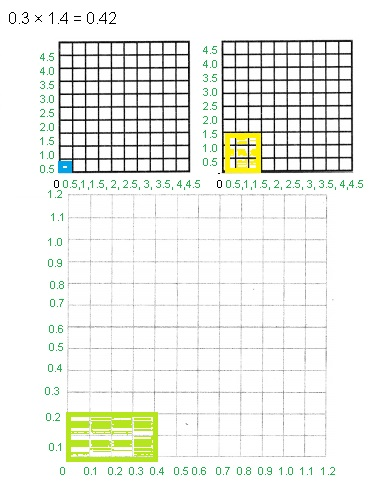Explanation:
Multiplying 0.3 × 1.4
STEP 1:

STEP 2:

Math Talk
Mathematical Processes

Explain why the product is less than only one of the decimal factors.
We are finding a fractional amount of a quantity,

Explanation:
When multiplying a number by a decimal less than one,
the product will be smaller than the number being multiplied.
This is because we are finding a fractional amount of a quantity.
For example, 0.1 x 0.8 = 0.08, because the question is asking us to
find one tenth of eight tenths.

Share and Show
.Multiply. Use the decimal model.

Question 1.
0.8 × 0.4 = ______0.32_______0.8 X 0.4 = 0.32,

Explanation:
Multiplying 0.8 × 0.4
STEP 1:

STEP 2:
Therefore rows of the shaded columns are 0.32.

Go Math Lesson 3.6 5th Grade Decimal Multiplication Models Question 2.
0.1 × 0.7 = _____0.07_______0.1 X 0.7 = 0.07,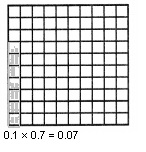Explanation:
Multiplying 0.1 × 0.7
STEP 1:

STEP 2:

Question 3.
0.4 × 1.6 = _____0.64_______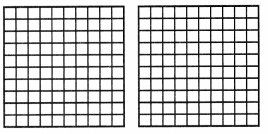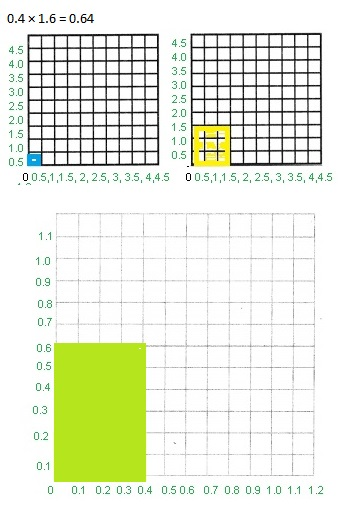Explanation:
Multiplying 0.4 × 1.6
STEP 1:

STEP 2:
Therefore rows of the shaded columns are 0.64.

Problem-Solving

Sense or Nonsense?

Model Decimal Multiplication 5th Grade Lesson 3.6 Question 4.
Use Diagrams Randy and Stacy used area models to find 0.3 of 0.5.
Both Randy’s and Stacy’s models are shown below.
Whose model makes sense? Whose model is nonsense?0.3 × 0.5 = __0.15__________
Randy’s model makes sense, Stacy’s model is nosense,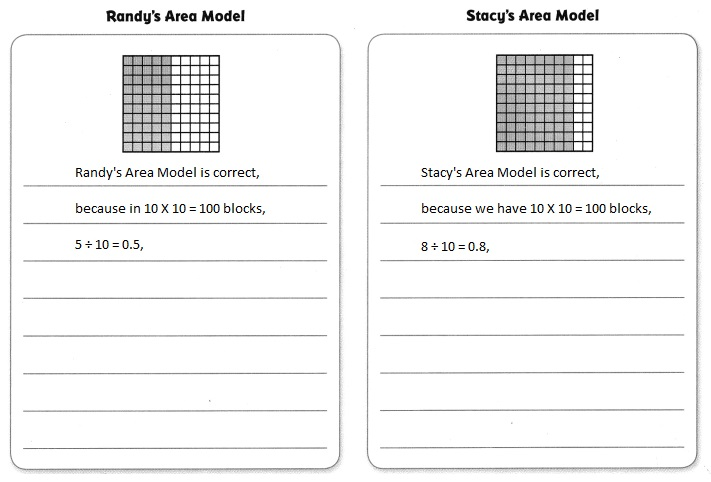0.3 X 0.5 = 0.15,

Explanation:
Given Randy and Stacy used area models to find 0.3 of 0.5.
Both Randy’s and Stacy’s models are shown above,
Randy’s model makes sense which matches with 0.5,
Stacy’s model is nosense as it doesnot match with 0.3.
the correct answer is 0.3 X 0.5 = 0.15.

Question 5.
H.O.T. Multi-Step A large bottle contains 1.2 liters of olive oil.
A medium-sized bottle has 0.6 times the amount of olive oil as the large bottle.
How much more olive oil does the large bottle contain than the medium-sized bottle?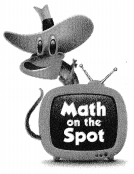0.48 liters more olive oil does the large bottle contain than the medium-sized bottle,

Explanation:
Given a large bottle contains 1.2 liters of olive oil,
A medium-sized bottle has 0.6 times the amount of olive oil as the large bottle,
therefore medium-sized bottle contains 1.2 liters X 0.6 = 0.72 liters,
Now more olive oil does the large bottle contain than the medium-sized bottle is
1.2 liters – 0.72 liters = 0.48 liters.

Question 6.
Representations Farmer Green grows 0.9 tons of grain each year.
Seven-tenths of the grain grown are barley. Which model shows
the amount of barley grown each year?Model (A) shows the amount of barley grown each year,

Explanation:
Given Farmer Green grows 0.9 tons of grain each year.
Seven-tenths of the grain grown is barley,
So farmer grows each year is 0.9 X 100 = 90 grains and
seven-tenth is 90 X 7÷ 10 = 63, the model showing
the amount of barley grown each year is 63 matches with (A).

Go Math Grade 5 Lesson 3.6 Decimal Model Multiplication Question 7.
Use Diagrams What multiplication problem is modeled by the diagram?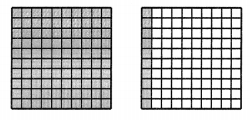(A) 1.3 × 0.3 = 1.03
(B) 0.7 × 0.3 = 1.03
(C) 1.1 × 0.3 = 0.3
(D) 0.3 × 1.1 = 0.33
(C) 1.1 × 0.3 = 0.3,

Explanation:
Given the multiplication problem modeled by the diagram as
frame 1- 10 X 10 = 100 ÷ 100 = 1,
and frame 2 as 1 X 10 ÷ 100 = 0.1,
therefore result in matches with (C) 1.1 × 0.3 = 0.3.

Question 8.
Multi-Step An outdoor swimming race is 1.6 miles long.
Simon swims 8 tenths of the course and his friend
Sheila swims 9 tenths of the course.
How much farther did Sheila swim?
(A) 0.16 mile
(B) 0.72 mile
(C) 0.1 mile
(D) 0.2 mile
(C) 0.1 mile,

Explanation:
Given an outdoor swimming race is 1.6 miles long,
Simon swims 8 tenths of the course means 8÷ 10 = 0.8 and
his friendSheila swims 9 tenths of the course so 9 ÷ 10 = 0.9,
So much farther did Sheila swims is 0.9 – 0.8 = 0.1 which matches with (C).

Texas Test Prep

Question 9.
Alex is 1.3 meters tall. His brother, Raul, is 0.6 times as tall as Alex. How tall is Raul?
(A) 1.9 meters
(B) 0.7 meter
(C) 0.78 meter
(D) 7.8 meters
(C) 0.78 meter,

Explanation:
Given Alex is 1.3 meters tall. His brother Raul is 0.6 times as tall as Alex.
Therefore Raul is 1.3 X 0.6 = 0.78 meters matches with (C).

### Texas Go Math Grade 5 Lesson 3.6 Homework and Practice Answer Key

Multiply. Use the decimal model.

Question 1.
0.6 × 0.9 = ___0.54______0.6 X 0.9 =Explanation:
Multiplying 0.6 × 0.7
STEP 1:

STEP 2:

0.5 × 1.7 = ___0.85_______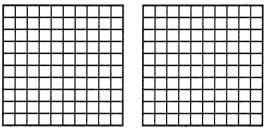Explanation:
Multiplying 0.5 × 1.7
STEP 1:

STEP 2:

Question 3.
0.2 × 0.8 = ____0.16________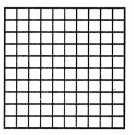0.2 X 0.8 = 0.16,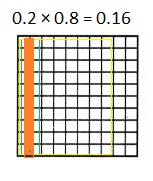Explanation:
Multiplying 0.2 × 0.8
STEP 1:

STEP 2:

Question 4.
0.3 × 0.7 = _____0.21_______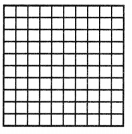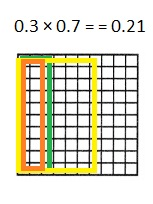Explanation:
Multiplying 0.3 × 0.7
STEP 1:

STEP 2:
Therefore rows of the shaded columns are 0.21.

Go Math Lesson 3.6 5th Grade Modeling Decimal Multiplication Question 5.
0.7 × 1.2 = _____0.84________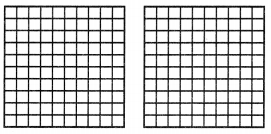0.7 X 1.2 = 0.84,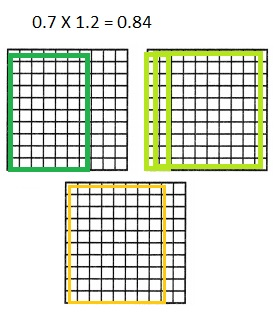Explanation:
Multiplying 0.7 × 1.2
STEP 1:

STEP 2:

Question 6.
0.4 × 0.6 = ______0.24_______0.4 X 0.6 = 0.24,Explanation:
Multiplying 0.4 × 0.6
STEP 1:

STEP 2:

Question 7.
0.8 × 0.8 = ______0.64_______0.8 X 0.8 = 0.64,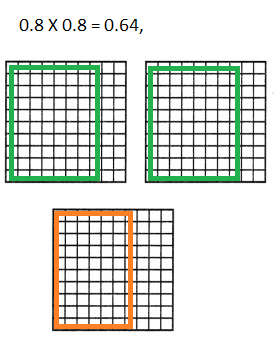Explanation:
Multiplying 0.8 × 0.8
STEP 1:

STEP 2:

Question 8.
0.4 × 1.9 = ___0.76__________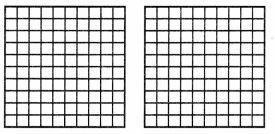0.4 X 1.9 = 0.76,Explanation:
Multiplying 0.4 × 1.9
STEP 1:

STEP 2:
Therefore rows of the shaded columns are 0.76.

Go Math Lesson 3.6 5th Grade Decimal Subtraction Question 9.
0.9 × 0.7 = _____0.63________0.9 X 0.7 = 0.63,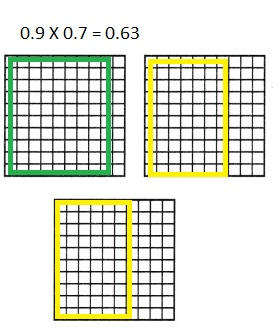Explanation:
Multiplying 0.9 × 0.7
STEP 1:

STEP 2:

Problem Solving

Question 10.
Nicole draws a mural on her wall. She begins by drawing a line that is 0.5 meter long.
She traces 2 tenths of the line with a purple pencil.
What is the length of the purple part of the line? Use the decimal model to solve.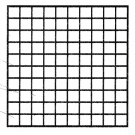Explanation:
Given Nicole draws a mural on her wall. She begins by drawing a line that is 0.5 meter long.
She traces 2 tenths of the line with a purple pencil.
So, the length of the purple part of the line is 0.5 X 0.2 = 0.10,
Shown using the decimal model to solve the given length of the
purple part of the line.

Question 11.
Vanesh uses 1.6 gallons of paint for his bedroom walls.
For his study, he uses 0.7 times
the amount of paint as he uses for his bedroom.
How much paint does Vanesh use to paint his study?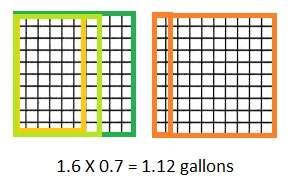Explanation:
Given Vanesh uses 1.6 gallons of paint for his bedroom walls.
For his study, he uses 0.7 times the amount of paint as he uses for
his bedroom is 1.6 X 0.7 = 1.12 gallons of paint Vanesh used to paint his study.

Lesson Check

Question 12.
Alex’s tablet computer weighs 1.5 pounds. The weight of his calculator is 0.6 times
the weight of his tablet computer. What is the weight of Alex’s calculator?
(A) 2.1 pounds
(B) 1.56 pounds
(C) 0.3 pound
(D) 0.9 pound
(D) 0.9 pound,

Explanation:
Given Alex’s tablet computer weighs 1.5 pounds. The weight of his calculator is 0.6 times
the weight of his tablet computer. So the weight of Alex’s
calculator is 1.5 pounds X 0.6 = 0.9 pound matches with (D).

Question 13.
Which equation is represented by the model below?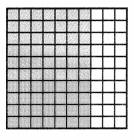(A) 0.1 × 0.7 = 0.07
(B) 0.7 × 0.6 = 0.42
(C) 0.6 × 0.2 = 0.12
(D) 6.0 × 7.0 = 42.0
(A) 0.1 × 0.7 = 0.07,

Explanation:
Given model above is 0.1 X 0.1 = 0.01,
So each block is 0.1 and we have 7 blocks like that
0.1 X 0.7 = 0.07 which matches with 0.07.

Question 14.
Sasha’s hundredths decimal model shows the product 0.72.
what factor represents the shaded rows?
(A) 0.6
(B) 0.9
(C) 0.7
(D) 0.8
(D) 0.8,

Explanation:
Given Sasha’s hundredths decimal model shows the product 0.72.
If she shaded 9 columns factor represents
the shaded rowsis 0.72 ÷ 0.9 = 0.8 matches with (D).

Question 15.
The postman delivers a package and an envelope to Mr. O’Connor.
The package weighs 1.8 pounds. The envelope is 0.8 times the weight of the package.
What is the weight of the envelope?
(A) 1.44 pounds
(B) 1 pound
(C) 1.16 pounds
(D) 1.64 pounds
(A) 1.44 pounds,

Explanation:
Given the postman delivers a package and an envelope to Mr. O’Connor.
The package weighs 1.8 pounds. The envelope is 0.8 times the weight of the package.
The weight of the envelope is 1.8 pounds X 0.8 = 1.44 pounds matches with (A).

Question 16.
Multi-Step Carter solves 0.5 × 0.5. Liam solves 0.4 × 1.4.
How much greater is Liam’s product than Carter’s product?
(A) 0.31
(B) 0.56
(C) 0.25
(D) 0.81
(A) 0.31,

Explanation:
Given Carter solved 0.5 X 0.5 as = 0.25 and Liam solved greater as
0.4 X 1.4 = 0.56, Greater is Liam’s product than Carter’s product is
0.56 – 0.25 = 0.31 matches with (A).

Question 17.
Multi-Step Jessica makes punch for her party. She uses 1.6 liters of orange juice.
The amount of cranberry juice she uses is 0.5 times the amount of orange juice, and
the amount of apple juice is 0.5 times the amount of cranberry juice.
What is the total amount of juice in Jessica’s punch?
(A) 2.6 liters
(B) 2.65 liters
(C) 2.8 liters
(D) 1.8 liters